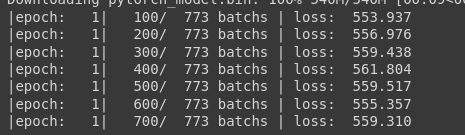# Training loss remained almost unchanged during training

Hi.
I am training a model.
This is my code to train the model.

``````    optimizer = optim.SGD(model.parameters(), lr=args.learning_rate)
criterion = InfoNCELoss(temperature=0.1)
scheduler = optim.lr_scheduler.StepLR(optimizer, 1.0, gamma=0.1)

log_iter = 100

for e in range(1, args.epoch + 1):
model.train()
t0 = time.time()
total_train_loss = 0

sentence1_embedding, sentence2_embedding = model(batch["sentence1"], batch["sentence2"])
train_batch_loss = criterion(sentence1_embedding, sentence2_embedding, batch["sentence1_label"], batch["sentence2_label"])

total_train_loss += train_batch_loss.item()
train_batch_loss.backward()
optimizer.step()
scheduler.step()
``````

This is my training loss:Training loss remained almost unchanged during training.